﻿

### Anote on elimination of imaginaries for pairs of fields

#### Abstract

We show that, if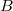$B$ is a field and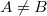$A \neq B$ is any algebraically closed  field extension of$B$, then the theory of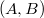$(A,B)$ eliminates imaginaries if  and only if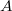$A$ is a finite extension of$B$ (and so if and only if$(A,B)$  is elementary equivalent to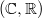$( \mathbb{C}, \mathbb{R})$).

DOI Code: 10.1285/i15900932v22n1p89

Keywords: Elimination of imaginaries; Pair of fields

Full Text: PDF